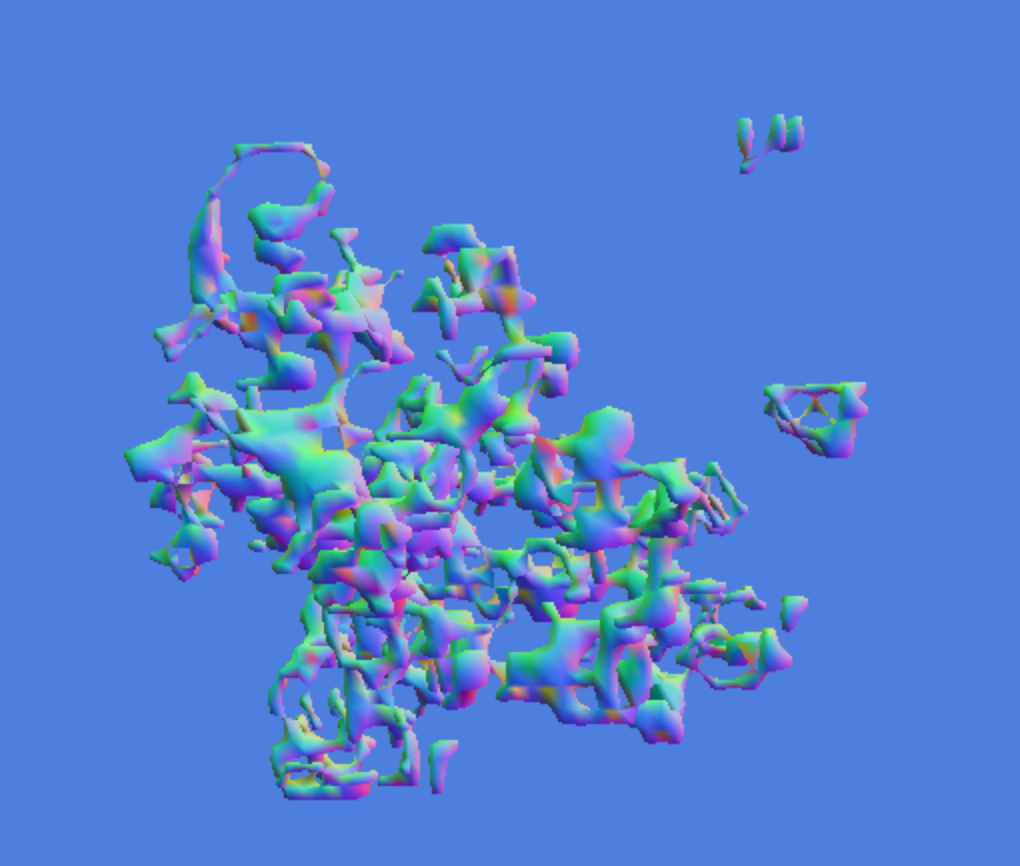Nobody's Perfect, Man

# npm

Have ideas to improve npm?Join in the discussion! »

## rle-funcs

0.0.6 • Public • Published

# rle-funcs

Basic primitives for merging and combining multiphase solid objects. This is part of the rle narrowband level set library.

# Installation

You can install it via rle-funcs:

``````npm install rle-funcs
``````

# Example

Here is an example showing how to implement the update rule for a 3D version of the Game of Life using narrowband level sets:

``````//Bounds for birth
var SURVIVE_LO      = 4;
var SURVIVE_HI      = 5;
var BIRTH_LO        = 5;
var BIRTH_HI        = 5;

//Set up neighborhood stencil
var MOORE_1         = require("rle-stencil").moore(1);
var CENTER_INDEX    = 13;

//Compute the next state of the cellular automaton
function nextState(state) {
return require("rle-funcs").apply(state, MOORE_1, function(phases, distances, retval) {
//Count neighbors
var neighbors = 0;
for(var i=0; i<27; ++i) {
if(i !== CENTER_INDEX && phases[i]) {
++neighbors;
}
}
//Compute next state
if(phases[CENTER_INDEX]) {
if(SURVIVE_LO <= neighbors && neighbors <= SURVIVE_HI) {
retval = 1;
return;
}
} else if(BIRTH_LO <= neighbors && neighbors <= BIRTH_HI) {
retval = 1;
return;
}
retval = 0;
return;
});
}

//Initialize state and tick in your main loop
``````

If you want to see it in action, here is a demo. Other examples of using rle-funcs can be found in rle-csg and rle-morphology which are built on top of this library:# Usage

## `merge(volumes, stencil, merge_func)`

This merges a collection of volumes together. This acts like a generalized boolean operation amongst the volumes. It is a very flexible, and very general function

• `volumes`: An array of volumes
• `stencil`: A stencil
• `merge_func(phases, distances, retval)`: A function that takes 3 arguments:
• `phases`: An array of length `volumes.length * stencil.length` of material phases.
• `distances`: An array of length `volumes.length * stencil.length` of distances to the material boundary
• `retval`: A length two array for the return value of the function. The first item is the new phase and the second item is the distance to the phase boundary.

Returns: A new volume which is the result of calling merge_func at every point in the volumes.

## `mergePoint(volumes, merge_func)`

This is an optimized version of merge where the stencil is a single point.

## `apply(volume, stencil, merge_func)`

This is an optimized version of `merge` that takes only a single volume as input, instead of an array. Useful for implementing cellular automata and other local differential equations.

## `applyPoint(volume, merge_func)`

Optimized version `apply` that assumes `stencil` is a single point.

# Credits

(c) 2013 Mikola Lysenko

## Keywords

none

### Install

`npm i rle-funcs`

4

0.0.6